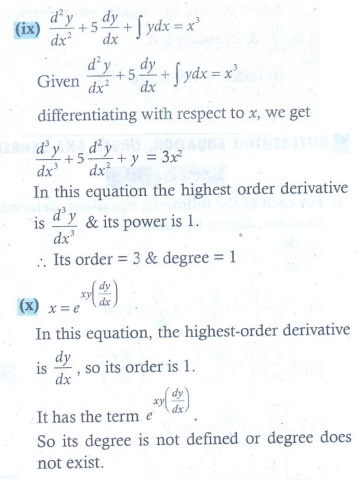Home | | Maths 12th Std | Exercise 10.1: Differential Equation, Order, and Degree

# Exercise 10.1: Differential Equation, Order, and Degree

Maths Book back answers and solution for Exercise questions - Mathematics : Ordinary Differential Equations: Differential Equation, Order, and Degree - Exercise Problem Questions with Answer, Solution

EXERCISE 10.1

1. For each of the following differential equations, determine its order, degree (if exists)